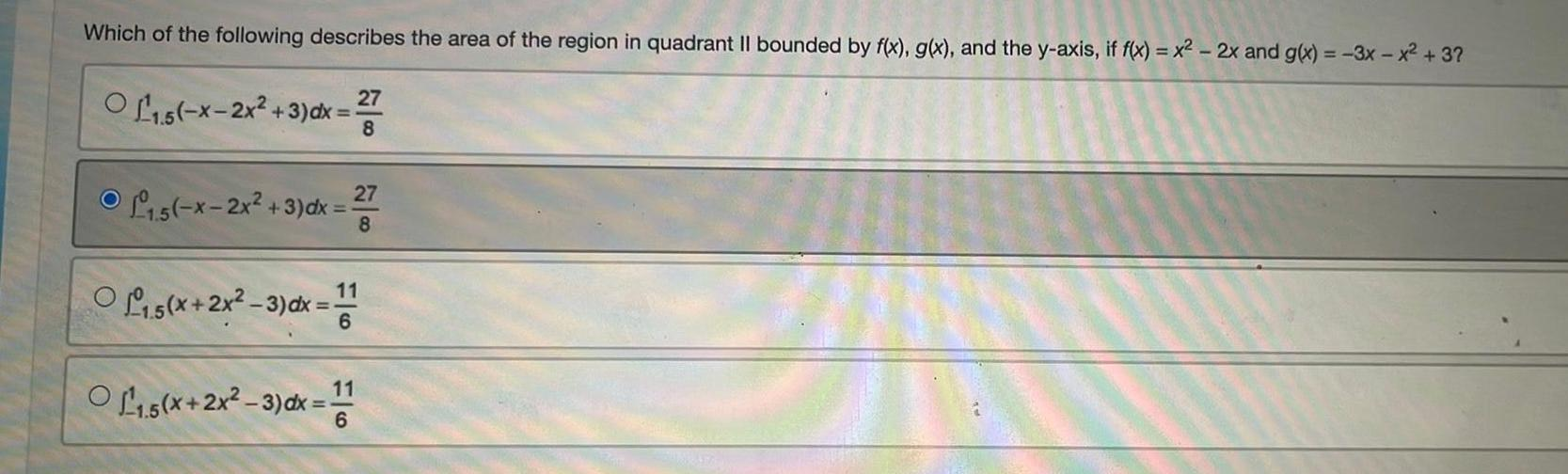Question:

# Which of the following describes the area of the region in

Last updated: 8/14/2022Which of the following describes the area of the region in quadrant II bounded by f(x), g(x), and the y-axis, if f(x) = x2 - 2x and g(x) = -3x - x² + 3?